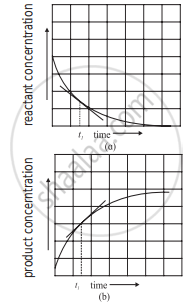Advertisement Remove all ads

# Answer the following in brief. How instantaneous rate of reaction is determined? - Chemistry

Answer in Brief

Answer the following in brief.

How instantaneous rate of reaction is determined?

Advertisement Remove all ads

#### Solution

i. To determine the instantaneous rate of a reaction the progress of a reaction is followed by measuring the concentrations of a reactant or product for different time intervals.

ii. The concentration of a reactant or a product is plotted against time.

iii. A tangent drawn to the curve at time t1 gives the rate of the reaction. The slope thus obtained gives the instantaneous rate of the reaction at time t1.Determination of instantaneous rate

iv. Mathematically the instantaneous rate is expressed by replacing Δ by derivative dc/dt in the expression of the average rate.

Instantaneous rate = ("d"["C"])/"dt"

v. For a reaction A → B

Instantaneous rate of consumption of A at time t = -("d"["A"])/"dt"

Instantaneous rate of the formation of B at time t = ("d"["B"])/"dt"

Therefore, instantaneous rate of the reaction at time t = -("d"["A"])/"dt" = ("d"["B"])/"dt"

Is there an error in this question or solution?
Advertisement Remove all ads

#### APPEARS IN

Balbharati Chemistry 12th Standard HSC for Maharashtra State Board
Chapter 6 Chemical Kinetics
Exercise | Q 3.01 | Page 136
Advertisement Remove all ads
Advertisement Remove all ads
Share
Notifications

View all notifications

Forgot password?